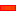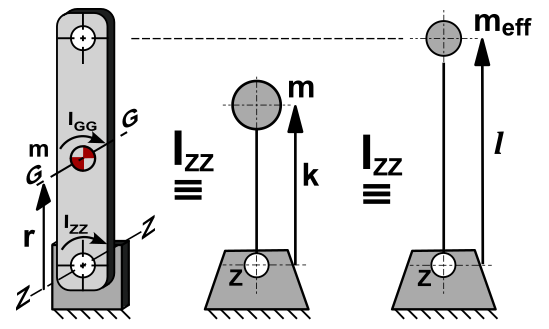This topic:

 • How we can normalize Payload Types with coefficients: Q, K, Cm
 • Reviews the effect on input-torque for different payloads types, and how we can normalize it as the coefficient, Cc

Notes on Terminology:

'Payload', also named 'load', is the total force, or torque,  referred to the cam-follower.

'Load' imposed on a cam-mechanism is also known as the duty of the mechanism.

'Capacity' is the ability of the mechanism to perform the duty for a specified lifetime.

###Payload: Load Types

It is important to know the payload-type when we design a cam-mechanism.

The output-load is passed from the cam-follower and back to the cam and the cam-shaft. Thus, the input-load that is imposed on a cam-shaft is a function of the load-type at the cam-follower.

The nominal output loading, which will be a Force for linear motion, and a Torque for rotary motion, is derived from the following 'Payload-Types'.A: Force or Torque: constant.

For example, friction, gravity, work loads.B: Force or Torque: proportional to displacement.

For example, a spring.C: Force or Torque: proportional to velocity.

For example, viscosity.D: Force or Torque: proportional to acceleration.

For example, inertia.E: Force or Torque: conforms to a special function.

For example, an engine cycle.

#### EXAMPLE 1A: Gravity, Spring and Inertia: Speed DependenceMECHANISM AT 'LOW-SPEED'

The two images in the example to the left show the same payload-types on a cam mechanism, at two different speeds.

The payload-types in the example are:

 • Constant ,
 • Spring , and
 • Inertia

You can see that at 'high-speed' the inertia is greater atthan the payload is at.  The phase at which the payload is a maximum is also different.

At the higher speed the load becomes negative at point, in the deceleration of the rise motion-segment.SAME MECHANISM AT 'HIGH-SPEED'

#### EXAMPLE 1B : Gravity, Spring and Inertia: Different Pre-load of Spring

The image below shows the period of negative loading when the cam has a 'falling' motion.

In such a case, the cam-follower would lose contact with an open track cam and control of the payload would be lost.

To make sure the cam-follower remains in contact with the cam-profile:

 • Improve the motion design to reduce the maximum negative acceleration.
 • Use an enclosed track, a blade cam, or a conjugate cam

- or -Virtually all indexing mechanisms, and many reciprocating and oscillating mechanisms, have a duty that combines a Constant (A) and an Acceleration (D) load, with less significant amounts from the other kinds of loads.

Constant Load - from friction forces (bearing seals), raising weights or gravitational force, and other work loads - can be resolved into a single force or torque resisting the output motion.

Acceleration Load - from the inertia of various masses - can be resolved into a single equivalent inertia connected to the output or the cam-follower.

The peak of the total load usually is at the same point as the maximum inertia load.

 • Index mechanisms: Friction(A) + Inertia(D).
 • Very high-speed mechanism: possibly significant viscous loading (C), and, of course, Inertia(D).

The maximum value and proportion of each type OF load component (A, B, C, D and E), depends on the application. Thus, there is an infinite number of load patterns. It is possible to tabulate normalized values for the most common combinations.

The idealised design of Conjugate Cams eliminates the need for a Spring to maintain the contact of the roller against the cam. Frequently, the cam-follower rollers are adjusted, or designed, to give an 'optimal' pre-load against the cam, so that the rollers do not slip.

This is an 'idealised design'. More frequently, manufacturing and machining tolerances, mean it is difficult to guarantee contact between the cam and the roller throughout the cam's rotation.

The roller will often lose contact during some part of the machine cycle.

Frequently, Conjugate Cams use a spring, air-cylinder or hydraulic device, to force one of the cam-follower rollers against the cam to eliminate backlash. The loading device only needs a short travel to compensate for manufacturing tolerances.

###Normalized Loads

The maximum inertia load is: [Using these simple equations: F= M.a ; T=I.αandThe Inertia Load Fi or Mi are 'nominal', and must be modified by the application of the Torsion-Factor, Ct.

A Constant Load, such as friction, is:

 •for linear motion
 •for rotary motion

When there is an output force component that is proportional to displacement, such as a spring force, it is convenient to add the maximum value spring force to the Friction-Force, Ff , or Friction-Moment, Mf , as if it were a constant load.

In that case, the total output-load calculation is conservative. You can take these as safe values for preliminary designs.

#### Load Mix: Inertia; Friction; Spring

Thus:Ct

###Pre-load Coefficient (K); Inertia Ratio (Q).Example Loads The Spring Rate Effect is defined by a 'pre-load coefficient', K. It is applicable to all cam systems. K is the ratio of minimum non-inertia loading : maximum non-inertia loading. Fp, Mp = minimum non-inertia load (p = pre-load) Ff, Mf  = maximum non-inertia load (f = full-load) Linear SystemRotary SystemWhen there is no spring load component (see top image to the left), Mf = Mp Thus,#### Inertia Ratio, Q

 The other coefficient we need to evaluate Cm, is the ratio of Peak Inertia : Maximum Non-Inertia loading. The Inertia Ratio, Q:orThe instantaneous output load for linear and rotary motions are:It follows that the maximum value of these is where:for both Linear and Rotary loading. Cm can be evaluated for a range of values of Q [range of 0 to 1] and K [range of 0 to 1]. w  = instantaneous normalised displacement coefficient w'' = instantaneous normalised acceleration coefficient

###Input Torque Coefficient

The input torque [drive torque] varies throughout the motion. The variation is a function of the output load pattern and the motion-law.

Since the output load pattern varies with the mix of inertia load, spring load, and constant load, so too does the input torque.

For all cases, the mix is defined with the factors Q and K , as defined above.

For each motion-law, the instantaneous input torque can be expressed as a function of the instantaneous output load:for linear motionfor rotary motion

Where: :The instantaneous output load, fo or mo, is a function of the normalized motion-law factors, the load mix, and the payload.

Thus, the above equations can also be expressed as:- or -The maximum value ofoccurs during the acceleration phase.

It can be simply expressed by introducing a normalized Input-Torque Coefficient, Cc.

Thus:for linear motionfor a rotary motion.

Mc

= Peak Input Torque

Cc = the maximum of:The value of Cc, the Input Torque Coefficient, can be evaluated for any motion-law and a range of load mix factors. The input torque patterns for some standard motion-laws are shown (below) for different values of Q and when K=1 [no spring loading]. From these patterns it can be seen that there is a wide disparity between the input torques of different motion-laws, for all values of Q.

#### Input Torque Coefficient, Cc [K= 1, and Q= 0 – 1]Drive Torque Fluctuations with each Motion-Law.

It is common to use the maximum value of the Input Torque Coefficient, Cc, when the inertia ratio, Q=1

Simple Harmonic Motion varies smoothly and gradually from a low positive peak to a moderate negative: this is a good characteristic.

However, if this motion-law is preceded or followed by a dwell, there will be a discontinuity of acceleration at the start and end of the motion, and the dynamic response may be unacceptable.• When the Inertia Ratio is 1, the input torque of the Modified-Trapezoid varies rapidly from a high positive to a low negative value at cross-over [X=0.5]:
 • A rapid reversal of Torque is bad because the input power source [motor] reaches a high drive torque which must rapidly to high braking torque. This might cause severe overrun if the input transmission has low inertia. Over-run means the drive speed is greater than the nominal drive speed of the cam-shaft.
 • If the input has a low rigidity, the drive shaft will suddenly 'unwind' to reverse its direction of 'twist'. This can cause input speed-oscillation of the cam-shaft.• When the Inertia Ratio is 1, the input torque of the Cycloidal varies more gradually than the Mod-Trap motion-law , however, not as gradually as the Mod-Sine, and it also has a higher peak value than the Mod-Sine motion-law.• When the Inertia Ratio is 1, the input torque of the Mod-Sine varies gradually from positive to negative.
 • It varies more gradually than, and is better than, Cycloidal.

This makes the Mod-Sine very tolerant of a bad input transmission which, combined with its good dynamic response properties, is the reason for its popularity.This image shows the:

Peak Values of Input-Torque Coefficient Cc against Q , Inertia Ratio, for each motion-law for constant loading (K=1).

#### Input Torque Coefficient, Cc [K= 0–1 & Q = 0–1]

The more complex loading pattern that includes a spring load component (0<K<1) we can find that both peak output load represented by Cm and the peak input torque, represented by Cc, are considerably reduced with low pre-load ratios.Tutorial and Reference Help Files for MechDesigner and MotionDesigner 13.2 + © Machine, Mechanism, Motion and Cam Design Software by PSMotion Ltd